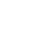# Python2.x中input（函数的漏洞）

``````# Python 2.x 程序显示两者之间的差异
# input() 和 rawinput() 函数
# 使用 raw_input() 函数的 3 个输入，
# 之后显示输入值的数据类型
s1 = raw_input("Enter input to test raw_input() function: ")
print type(s1)
s2 = raw_input("Enter input to test raw_input() function: ")
print type(s2)
s3 = raw_input("Enter input to test raw_input() function: ")
print type(s3)
# 使用 input() 函数的 3 个输入，
# 之后显示输入值的数据类型
s4 = input("Enter input to test input() function: ")
print type(s4)
s5 = input("Enter input to test input() function: ")
print type(s5)
s6 = input("Enter input to test input() function: ")
print type(s6)
``````

``````Hello
456
[1,2,3]
45
"goodbye"
[1,2,3]
``````

``````Enter input to test raw_input() function:
Enter input to test raw_input() function:
Enter input to test raw_input() function:
Enter input to test input() function:
Enter input to test input() function:
Enter input to test input() function:
``````

input() 方法中的漏洞

input() 方法的漏洞在于，任何人都可以通过使用变量或方法的名称来访问访问输入值的变量。让我们一一探讨：

Python 2.x 程序使用变量显示 input() 函数中的漏洞

``````import random
secret_number = random.randint(1,500)
print "Pick a number between 1 to 500"
while True:
res = input("Guess the number: ")
if res==secret_number:
print "You win"
break
else:
print "You lose"
continue
``````

Python 3 演示 input() 函数的差异

``````import random
secret_number = random.randint(1,500)
print ("Pick a number between 1 to 500")
while True:
res = input("Guess the number: ")
if res==secret_number:
print ("You win")
break
else:
print ("You lose")
continue
``````

``````15
``````

``````Pick a number between 1 to 500
Guess the number: You lose
Guess the number:
``````

``````secret_number
``````

``````Pick a number between 1 to 500
Guess the number: You win
``````

Python 3 显示了不同的结果。如果“secret_number”作为输入，答案是“You lose”。

``````# Python 2.x 程序通过传递函数名作为参数来演示 input() 函数漏洞
secret_value = 500
# 返回秘密值的函数
def secretfunction():
return secret_value
# 使用 raw_input() 输入数字
input1 = raw_input("Raw_input(): Guess secret number: ")
# input1 将被显式转换为字符串
if input1 == secret_value:
print "You guessed correct"
else:

# 使用 input() 输入数字
input2 = input("Input(): Guess the secret number: ")
# input2 在输入时进行评估
if input2 == secret_value:
print "You guessed correct"
else:
``````

``````400
secretfunction()
``````

``````Raw_input(): Guess secret number: wrong answer
Input(): Guess the secret number: You guessed correct
``````

``````secretfunction()
secret_value
``````

``````Raw_input(): Guess secret number: wrong answer
Input(): Guess the secret number: You guessed correct
``````

``````n = int(raw_input())
``````

《Python2.x中input（函数的漏洞）》